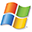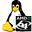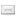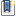ATOMS : Distfun details

# Distfun

Distribution functions
Details
Version
0.6
A more recent valid version with binaries for Scilab 5.4 exists: 0.9
Authors
Michael Baudin
Prateek Papriwal
Pierre Lecuyer
Luc Devroye
Jean-Philippe Chancelier
Michael A. Malcolm
Cleve B. Moler
George Marsaglia
Arif Zaman
Barry W. Brown
James Lovato
Kathy Russell
Makoto Matsumoto
Takuji Nishimura
Bruno Pincon
Richard Brent
John Burkardt
Owner Organization
INRIA, DIGITEO and others
Maintainers
Michael BAUDIN
prateek papriwal
Category
Dependencies
Creation Date
January 5, 2013
Source created on
Scilab 5.4.x
Binaries available on
Scilab 5.4.x:Linux 32-bitWindows 32-bitWindows 64-bitmacOSLinux 64-bit
Install command
`--> atomsInstall("distfun")`
Report a bugDescription
```            Distribution Functions toolbox

The goal of this toolbox is to provide accurate distribution functions.
The provided functions are designed to be compatible with Matlab.

The goals of this toolbox are the following.
* All functions are tested with tables (actually, csv datasets).
The tests includes accuracy tests, so that the accuracy
should by from 13 to 15 significant digits.
* For each distribution, we have
* the probability distribution function (PDF)
* the cumulated distribution function (CDF)
* the inverse CDF
* the random number generator
* the statistics (mean and variance)
* The CDF provides the upper and the lower tail of the
distribution, for accuracy reasons.
* The uniform random numbers are of high quality.
The default is to use the Mersenne-Twister generator.
* Each function has a consistent help page.
This removes confusions in the meaning
of the parameters and clarifies the differences
with other computing languages (e.g. R).

The design is similar to Matlab's distribution functions.
A significant difference with Matlab's function is that both
the upper and lower tails are available in "distfun", while
Matlab only provides the lower tail.
Hence, "distfun" should provide a better accuracy when
probabilities close to 1 are computed (e.g. p=0.9999).

There are many interesting, positive, differences with Scilab, Stixbox, or other

http://forge.scilab.org/index.php/p/distfun/

Features
--------

For each distribution x, we provide five functions :
* distfun_xcdf — x CDF
* distfun_xinv — x Inverse CDF
* distfun_xpdf — x PDF
* distfun_xrnd — x random numbers
* distfun_xstat — x mean and variance

Distributions available :
* Beta (with x=beta)
* Binomial (with x=bino)
* Chi-Square (with x=chi2)
* Exponential (with x=exp)
* F (with x=f)
* Gamma (with x=gam)
* Geometric (with x=geo)
* Hypergeometric (with x=hyge)
* LogNormal (with x=logn)
* Normal (with x=norm)
* Poisson (with x=poi)
* T (with x=t)
* Uniform (with x=unif)

Tutorial
* dispfun_tutorial — A tutorial of the Distfun toolbox.
* dispfun_plots — A collection of distribution function plots.

Support
* distfun_cov — Returns the empirical covariance matrix
* distfun_erfcinv — Inverse erfc function
* distfun_genericpdf — Compute the PDF from the CDF.
* distfun_getpath — Returns path of current module
* distfun_inthisto — Discrete histogram
* distfun_plotintcdf -  Plots an integer CDF

Random Number Generator
* rng_overview — An overview of the Random Number Generators of the Distfun
toolbox.
* distfun_genget — Get the current random number generator
* distfun_genset — Set the current random number generator
* distfun_seedget — Get the current state of the current random number
generator
* distfun_seedset — Set the current state of the current random number
generator
* distfun_streamget — Get the current stream
* distfun_streaminit — Initializes the current stream
* distfun_streamset — Set the current stream            ```Files (6)Source code archive
```
```Linux 32-bit binary for Scilab 5.4.x
```Linux 32-bit
Automatically generated by the ATOMS compilation chain

```Windows 32-bit binary for Scilab 5.4.x
```Windows 32-bit
Automatically generated by the ATOMS compilation chain

```Windows 64-bit binary for Scilab 5.4.x
```Windows 64-bit
Automatically generated by the ATOMS compilation chain

```macOS binary for Scilab 5.4.x
```MacOSX version
Automatically generated by the ATOMS compilation chain

```Linux 64-bit binary for Scilab 5.4.x
```Linux 64-bit
Automatically generated by the ATOMS compilation chain

```News (0)Comments (2)Comment from Michael BAUDIN-- January 5, 2013, 11:21:30 PM
```Here is the changelog.

distfun (0.6)
* Updated the order of the internal (private) random
generator C function to match the order of the
documented functions (no switching anymore).
* Updated the name of the internal (private) random
generator C function to match the name of the
documented functions (no "grand" anymore).
* Fixed bug #979.
distfun_fstat did not give the formula for M and V
* Fixed bug #978
Connected the exponential random variable generator to the gateway.
Created and connected a Log-Normal random variable generator.
Created and connected a T random variable generated.
Created and connected a Hypergeometric random variable gateway.
* Fixed bug #977
distfun_binornd failed for large N
* Fixed bug #982
distfun_poissrnd failed for large lambda
Also improved accuracy of constants in Poisson
random number generator.
* Fixed bug #986
distfun_gamrnd failed for small parameters.
* Fixed bug #989
Some tests fail on Linux 64 for v0.5.
Replaced the inversion routine with a clean C implementation of
Brent's algorithm (John Burkardt C code).
This also fix problems with the previous implementation, where the
number of iterations seemed to be large for some inputs.
* Created function distfun_verboseset.

```Comment from Michael BAUDIN-- January 11, 2013, 12:51:35 PM
```Here is a brief validation report.
The toolbox compiles and loads without problem on all systems.
All tests pass on Linux 32, Linux 64 and Windows 32.
Some tests (erfcinv, betacdf and betapdf) do not pass on Windows 64, and Mac 64 but this is

minor.
This release seems OK.```Leave a comment
Login withEmail notifications